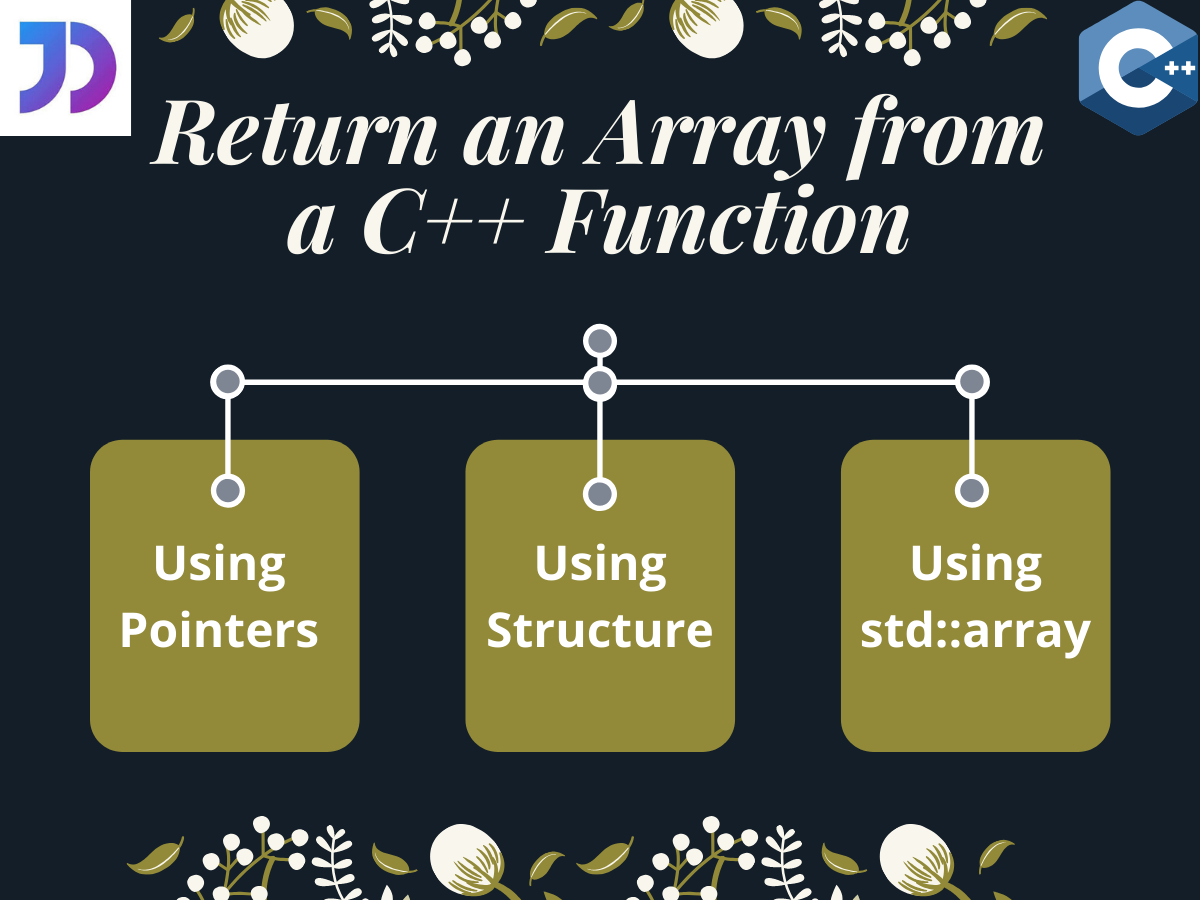# How to Return an Array in a C++ Function

Published on August 3, 2022By SnehWhile we believe that this content benefits our community, we have not yet thoroughly reviewed it. If you have any suggestions for improvements, please let us know by clicking the “report an issue“ button at the bottom of the tutorial.

## Introduction

In this tutorial, we are going to understand how we can return an array from a function in C++.

## Methods to Return an Array in a C++ Function

Typically, returning a whole array to a function call is not possible. We could only do it using pointers.

Moreover, declaring a function with a return type of a pointer and returning the address of a C type array in C++ doesn’t work for all cases. The compiler raises a warning for returning a local variable and even shows some abnormal behavior in the output.

Hence, returning an array from a function in C++ is not that easy. But we can accomplish that by following any of the below mentioned methods.

Let’s get right into it.

### 1. Using Pointers

As we mentioned earlier, returning a normal array from a function using pointers sometimes gives us unexpected results. But this behaviour and warnings can be avoided by declaring the array to be a `static` one.

Let us see how.

``````#include<iostream>
using namespace std;

int* demo() //return type- address of integer array
{
static int a; //array declared as static
for(int i = 0; i<5; i++)
{
a[i] = i; //array initialisation
}

return a; //address of a returned
}

int main()
{
int* ptr; //pointer to hold address
int i;
ptr = demo(); //address of a
cout<<"Array is: ";
for(i=0 ; i<5; i++)
cout<<ptr[i]<<"\t"; //ptr[i] is equivalent to *(ptr+i)

return 0;
}
``````

Output:

``````Array is: 0     1       2       3       4
``````

Here, we have declared the function `demo()` with a return type `int *`(pointer) and in its definition, we have returned `a` (serves as both array name and base address) to site of the function call in `main()`.

As we can see from the above output, the array is successfully returned by the function.

### 2. Using a Structure in C++

We can also make a function return an array by declaring it inside a structure in C++. Let us see how.

``````#include <iostream>
using namespace std;

struct demo
{
//array declared inside structure
int arr;
};

struct demo func(int n) //return type is struct demo
{
struct demo demo_mem; //demo structure member declared
for(int i=0;i<n;i++)
{
//array initialisation
demo_mem.arr[i] = i;
}
return demo_mem; //address of structure member returned
}

int main()
{
struct demo a;
int n=5; //number of elements

cout<<"The Array is : ";
for(int i=0;i<n;i++)
{
cout<<a.arr[i]<<"\t";
}

return 0;
}
``````

Output:

``````Array is: 0     1       2       3       4
``````

Here, note that we have declared the array `arr` inside the structure `demo`. And this time the function has a return type of the structure itself and return `demo_mem` (structure variable) instead of the array.

In this way using another structure variable `a`, we can access the array `arr` in the `main()` function.

### 3. Using std::array

For `std::array` in C++, returning the array name from a function actually translates into the the whole array being returned to the site of the function call.

``````#include <iostream>
#include<array>
using namespace std;

std::array<int,5> func() //function with return type std::array
{
std::array<int,5> f_array; //array declared

for(int i=0;i<5;i++)
{
//array initialisation
f_array[i] = i;
}

return f_array; //array returned
}

int main()
{
std::array<int,5> arr; //array with length 5

arr=func(); //function call

cout<<"The Array is : ";
for(int i=0;i<5;i++)
{
cout<<arr[i]<<"\t";
}

return 0;
}
``````

Output:

``````Array is: 0     1       2       3       4
``````

Hence it is clear from the output, that the array return by the function `func()` was successful.

## Conclusion

So in this tutorial, we learned about the different methods by which we can return an array from a C++ function.

For any further questions, feel free to use the comments below.

Click below to sign up and get \$200 of credit to try our products over 60 days!

### Popular Topics

##### Join the Tech Talk##### Hollie's Hub for Good

Working on improving health and education, reducing inequality, and spurring economic growth? We’d like to help.